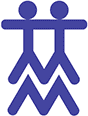# Math Materials

Montessori believed that a child’s mind is mathematical and based on the order of perceptual awareness found in the development of the senses.  The acquisition of mathematical principles is seen as developing logically from concrete to abstract, and from simple to complex.  The Montessori Math includes the development of concepts such as numeration, place value, fractions, and the basic operations of addition, subtraction, multiplication, and division using numbers up to 9999.Sandpaper Numbers

The math sequence begins with learning the names “one-ten” and being able to place the correct quantity (number) with the appropriate symbol (numeral).  Basic materials used for learning this concept are Number Rods, Sandpaper Numerals, Spindle Boxes, and Cards and Counters.Cards Counters

Once the child has learned to count to 10 and to place the correct quantity with each numeral, the child is able to move on to working with 9 units, 9 hundreds, 9 tens, and 9 thousands.  It is as easy to work with these hierarchical objects as it is to work with adding and subtracting apples, pears, oranges, and bananas.

Using these concrete objects, the child manipulates the materials and gains an understanding that addition means to join together, subtraction means to take away, multiplication means to join the same quantity many times, and division means to share equally.  The equipment used for learning this concept is called The Golden Bead Material or The Decimal system. After the child has become familiar with the Golden Bead Material and the function of each operation, the hierarchical values are presented: one thousand = 10 hundreds; 1 hundred = 10 tens; 1 ten = 10 units.  The child is now ready to use numeral cards to represent the quantities in each operation.  Exchanging (borrowing and carrying) are also practiced with these concrete materials.The child is also presented with the basic facts of the four operations, sometimes called the Tables.  Again, the concept is presented using concrete materials before moving into the abstract.  Here the child learns facts such as 1+1=2, 1+2=3, 1+3=4, 1+4=5, up to 1+9=10. Then the child moves on to 2+_; then 3+_; up to 9+9=18.  With a special board called the Strip Board, the child practices each addition table and also learns about the Commutative Principle (5+2=2+5). There are math materials for practicing the subtraction tables (18-9=9; 17-9=8, 17-8=9; etc, based on the reverse of the addition facts) and the multiplication basic facts going from 1×1=1 through all the multiplication tables to 10×10=100. With all of the work on the basic arithmetic facts, the child uses bead bars, numerous tables and charts, and strips with printed equations.  The concept of division is presented and practiced by sharing unit beads among up to 9 skittles.  Actually learning the division facts in an order is usually left to the future elementary grades.The child is also learning to write the numerals, first by feeling the Sandpaper Numerals, then tracing doted numerals, and finally writing numerals.

Halves, thirds, and fourths are also introduced, and the children learn first to identify these fractions and then to add and subtract fractions of like denominators.

By the time the child leaves the Montessori kindergarten program, he/she is well on the way of understanding our Base 10 system of numeration.